Courses

# Biot Savart Law, Amperes Law & Magnetic Force: Assignment Notes | EduRev

## IIT JAM : Biot Savart Law, Amperes Law & Magnetic Force: Assignment Notes | EduRev

The document Biot Savart Law, Amperes Law & Magnetic Force: Assignment Notes | EduRev is a part of the IIT JAM Course Electricity & Magnetism.
All you need of IIT JAM at this link: IIT JAM

Q.1. A square loop is placed near an infinite straight wire as shown in figure. The loop and wire carry a steady current I2 and I1 respectively. Then find the net force acting on the square loop.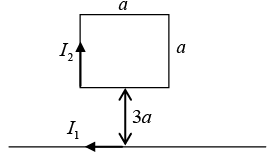The force on the two sides cancels.
At the bottom,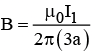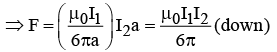At the top,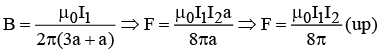Thus Net Force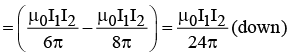Q.2. A system of two circular co-axial coils carrying equal currents I along same direction having equal radius R and separated by a distance R (as shown in the figure below). Find the magnitude of magnetic field at the midpoint P.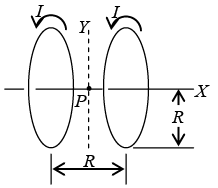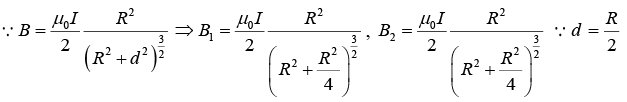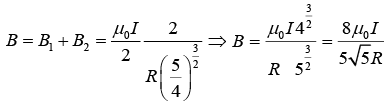Q.3. Two long conductors are arranged as shown below to form overlapping cylinders, each of radius R, whose centers are separated by a distance d. Current of density J flows into the plane of the page along the shaded part of one conductor and an equal current flows out of the plane of the page along the shaded portion of the other, as shown. What are the magnitude and direction of the magnetic field at point A ?

(Assumes that the vacuum region in the center is small compared to the area of the conductors)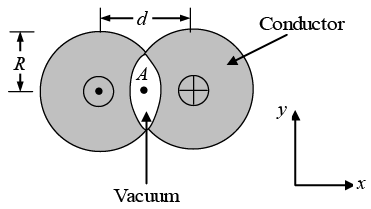Recall Ampere’s Law,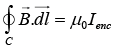Thus, the field from each conductor is B(2πr) = μ0JπR2, where, Ienc = JπRand R is the radius of the conductor.
(This is a good approximation of the current, as one assumes that the vacuum region in the center is small compared to the area of the conductors.)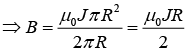Making the approximation that R ≈ d / 2, one has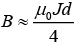Since both fields contribute in the center, the field is twice that,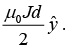Q.4. A conducting wire is in the shape of a regular hexagon, which is inscribed inside an imaginary circle of radius R, as shown in figure. A current I flows through the wire. Find the magnitude of the magnetic field at the center of the circle.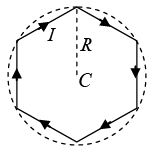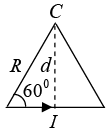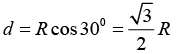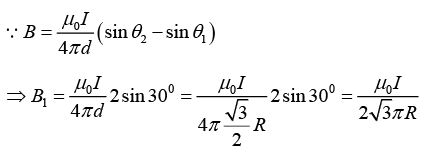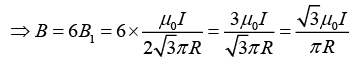Q.5. Consider two infinitely long wires parallel to the z -axis carrying the same current I. One wire passes through the point L with coordinates (-1,1) and the other through M with coordinates (-1,1) in the XY planes shown in the figure. The direction of the current in both the wires is in the positive z -direction.
(a) Find the value of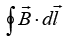along the semicircular closed path of radius 2 units shown in the figure.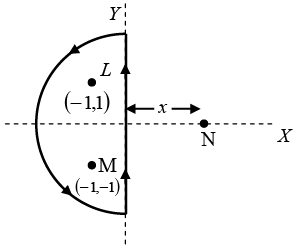(b) A third long wire carrying current I and also perpendicular to the XY plane is placed at a point N with coordinates (x,0) so that the magnetic field at the origin is doubled. Find x and the direction of the current in the third wire.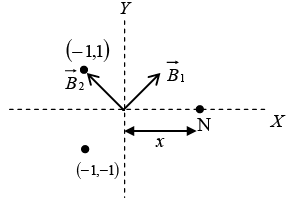(a)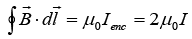(b)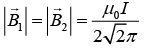Resultant of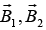is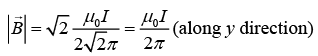The current in the third wire is inward so that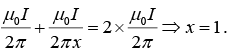Q.6. Find the magnitude and direction of a force vector acting on a unit length of a thin wire, carrying a current I = 8.0A, at a point O, if the wire is bent as shown in
(a) Figure (a), with curvature radius R = 10 cm;
(b) Figure (b), the distance between the long parallel segments of the wire being equal to
l = 20 cm.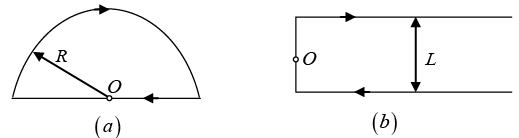(a) The magnetic field at O is only due to the curved path, as for the line element,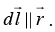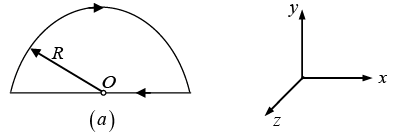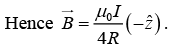Force per unit length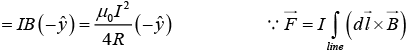Magnitude of force per unit length is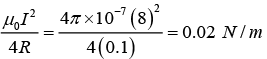(b) In this part, the magnetic field at O will be effective only due to semi infinite segments of wire.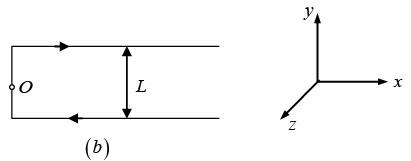Hence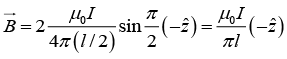Force per unit length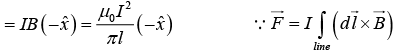Magnitude of force per unit length is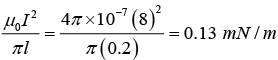Q.7. Three long, straight wires in the xz -plane, each carrying current I , cross at the origin of coordinates, as shown in the figure above. Let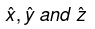denote the unit vectors in the x-, y- and z- directions, respectively. Find the magnetic field B as a function of x, with y = 0 and z = 0 .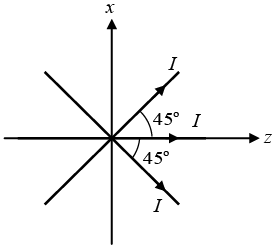Take a point ( x, 0, 0 ) on the x -axis.
At this point the magnetic field due to the wire along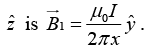Due to the wire crossing the first and third quadrants is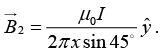Due to the wire crossing the second and fourth quadrants is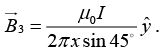Thus, the net magnetic field at point ( x, 0, 0 ) is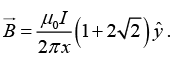Q.8. A ring of radius R carries a linear charge density λ and it is rotating with angular speed ω. Then
(a) Find the magnetic field at a distance R above its center.
(b) Find the magnetic field at its center.

(a)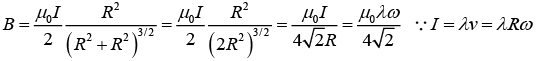(b)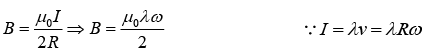Q.9. Consider a rectangular wire frame whose diagonal length is d and the angle between the diagonal is ϕ as shown in figure. If the current flowing in the frame is I, then the magnetic field B at the centre of the frame is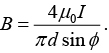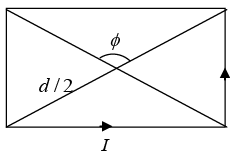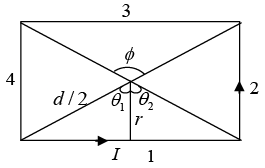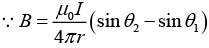where θ1 = -ϕ/2 and θ= ϕ/2
Magnetic field due to segment 1 and 3 is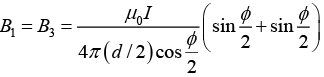Magnetic field due to segment 2 and 4 is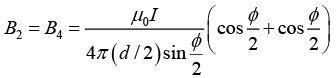Hence, the magnitude of total magnetic field is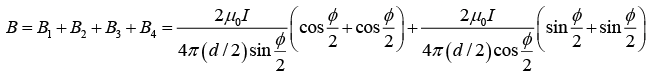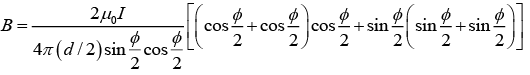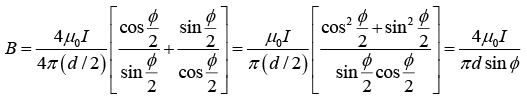Q.10. Three infinitely-long conductors carrying currents I1 ,I2 and I3 lie perpendicular to the plane of the paper as shown in the figure. If the value of the integral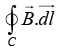for the loops C1 ,C2 and C3 are 2μ0 , 4μ0 and μ0 in the units of N/A respectively, then find I1 , Iand I3.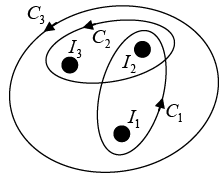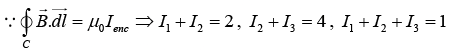⇒ I1 = -3A, I2 = 5A and I3 =-1A.
I1 = 3A into the paper, I2 = 5A out of the paper and I3 = 1A into the paper

Offer running on EduRev: Apply code STAYHOME200 to get INR 200 off on our premium plan EduRev Infinity!

## Electricity & Magnetism

2 videos|29 docs|22 tests

,

,

,

,

,

,

,

,

,

,

,

,

,

,

,

,

,

,

,

,

,

,

,

,

;